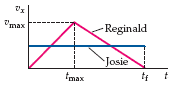# Relationship Between Velocities of Two Runners

Staff Emeritus
2022 Award

## Homework Statement

Reginald is out for a morning jog, and during the course of his run on a straight track, he has a velocity that depends upon time as shown in the figure below. That is, he begins at rest, and ends at rest, peaking at a maximum velocity Vmax at an arbitrary time tmax. A second runner, Josie, runs throughout the time interval t = 0 to t = t f at a constant speed Vj, so that each has the same displacement during the time interval. Note: t f is NOT twice t max , but represents an arbitrary time. What is relation between Vj and tmax?

## Homework Equations

Position equation for constant acceleration: X=Xi+Vi+1/2AT2
Velocity Equation for constant acceleration: V=Vi+AT
Position Equation for constant velocity: X=Xi+VT3. The Attempt at a Solution

Not sure what to do really, so I've just been trying things.
The only commonality between the two runners is the final position X and the time tf

For Josie, X = Vjtf

For Reginald:
Velocity:
From ti to tmax, Vmax = Atmax
From tmax to tf, since Vf = 0, the equation is: 0 = Vmax + Atf

Position:
From ti to tmax, initial displacement and velocity are zero: X1 = 1/2Atmax2
From tmax to tf: X = X1 + Vmaxtf + 1/2Atf2

Since X = Vjtf, we can rewrite the above equation as: Vjtf = X1 + Vmaxtf + Atf2
Replacing X1 with its equation: Vjtf = 1/2Atmax2+ Vmaxtf + Atf2

That's about as far as I've gotten and I don't know if I'm even on the right track.

Edit: Just so I don't look like an idiot, the question I posted was a literal copy and paste. I didn't just misread Vmax as Tmax.